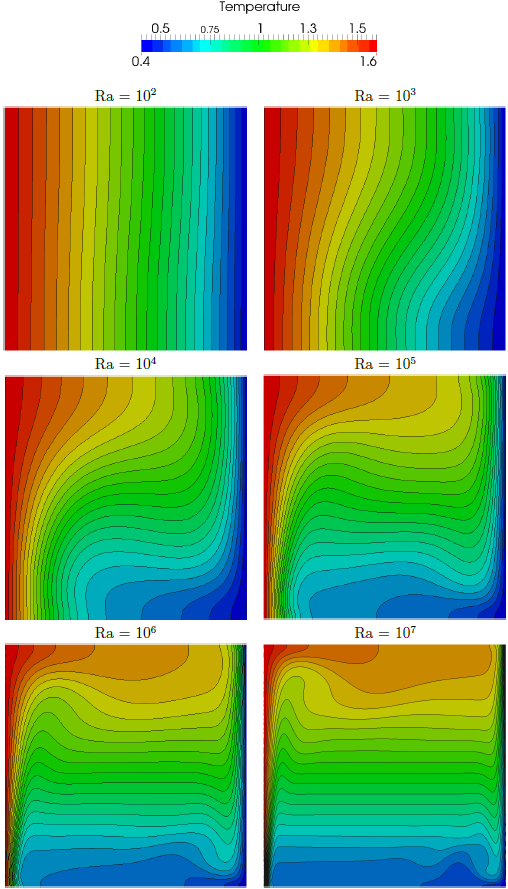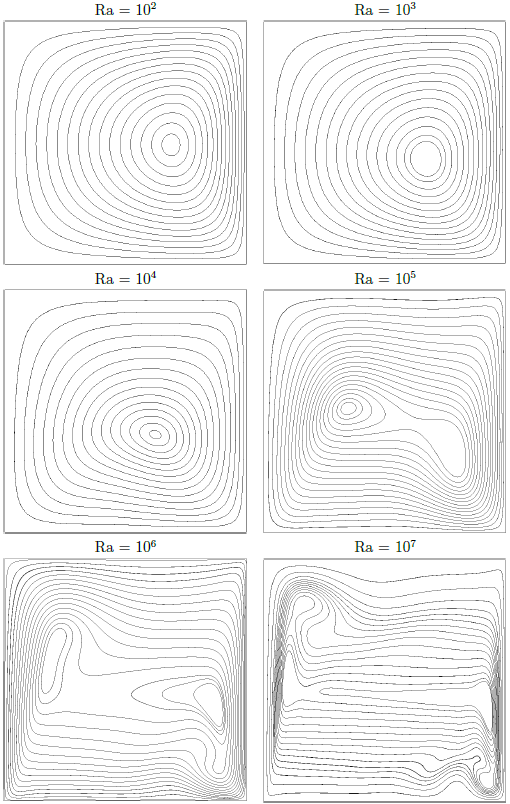# Low-Mach equations# Low-Mach number flows

The solver for non-isothermal flows is based on the low-Mach number equations, i.e. the density is a function of the thermodynamic pressure (which is constant in space) and the temperature but does not depend on the hydrodynamic pressure. A segregated approach based on the SIMPLE algorithm is used to solve the equations for the primitive variables in each time step. A mixed-order formulation is used for the spatial DG discretization, i.e. the polynomial order for the pressure is reduced by one compared to the order for the velocity and the temperature.

# Convected density jump

by: Benedikt Klein

The first test case for the variable density solver is the convection of a smooth density jump, which can also be seen as a multiphase problem with a smooth interface. In this case, the density is a linear function of a conservative level set which is advected with the velocity of the flow field. A fairly high density ratio of 1000 is applied. The h-convergence study for the level set function presented below shows a convergence rate of k+1.Density profile of a convected density jump on a Cartesian grid with 8 x 40 cells and polynomial degrees of 3, 3 and 2 for velocity, level set and pressure, respectively.

# Couette flow with temperature gradient

by: Benedikt Klein

For Couette flow with a cold temperature Tc applied to the lower wall and a hot temperature Th to the upper wall, the analytical solution

\begin{align*}& u = x_2,\\& p = -\frac{p_0}{Fr^2(T_h – T_c)} \ln\left((T_h – T_c) x_2 + T_c\right) + C,\\& T = (T_h – T_c) x_2 + T_c\end{align*}

can be derived, where constant viscosity and heat conductivity have been assumed. The h-convergence study shown below on successively refined Cartesian grids shows the high-order accuracy of the method.

Klein, Benedikt ; Müller, Björn ; Kummer, Florian ; Oberlack, Martin (2016):
A high-order Discontinuous Galerkin solver for low-Mach number flows.
81, In: International Journal for Numerical Methods in Fluids, (8), S. 489-520, ISSN 02712091, [Online-Edition: http://dx.doi.org/10.1002/fld.4193],
[Artikel]

# Natural convection in a heated cavity

by: Benedikt Klein

The natural convection in a square cavity is a common benchmark test for low-Mach number solvers. A hot temperature is applied to the left wall and a cold temperature to the right wall, while the upper and lower domain boundaries are adiabatic walls. The equations for low-Mach number flows are solved on a rather coarse uniform Cartesian grid with 26 x 26 cells. Polynomial orders are 5 for velocity and temperature and 4 for pressure. Below contour plots for the temperature and the streamlines are shown for various Rayleigh numbers ranging from Ra=102 to Ra=107.Temperature contours for natural convection in a heated cavity. Results are calculated on Cartesian grid with 26 x 26 cells and polynomial orders of 5 for velocity and temperature and 4 for pressure.Streamlines for natural convection in a heated cavity. Results are calculated on Cartesian grid with 26 x 26 cells and polynomial orders of 5 for velocity and temperature and 4 for pressure.

Klein, Benedikt ; Müller, Björn ; Kummer, Florian ; Oberlack, Martin (2016):
A high-order Discontinuous Galerkin solver for low-Mach number flows.
81, In: International Journal for Numerical Methods in Fluids, (8), S. 489-520, ISSN 02712091, [Online-Edition: http://dx.doi.org/10.1002/fld.4193],
[Artikel]